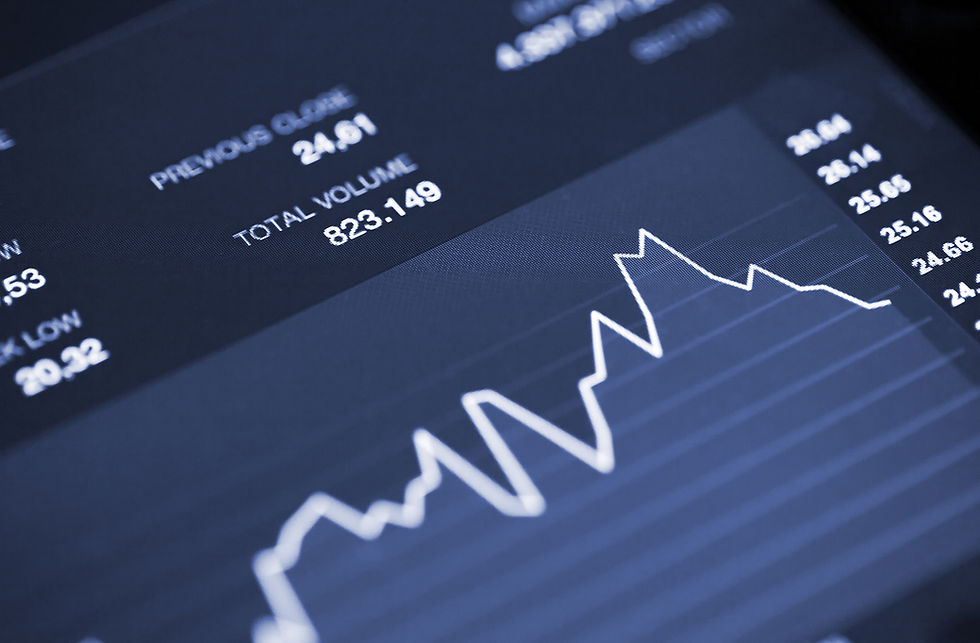top of page
Search

# Build Forecasted Analysis With Time Series Forecasting ModelHave you ever wished to predict the trend in stock price or the next pandemic could happen? Both situations required time to make the prediction. Time is the most important factor in building a time series model. For example, it would be interesting to forecast at what peak hour during the day trading is the highest, this would be crucial information to day traders.#### What are time-series forecasting models?

A time series forecasting model is a mathematical model that is used to predict future values of a time series based on past values. As the name suggests, forecasting: predicting the unknown values.

There are several reasons why companies adopt forecasting models, such as predicting future values, monthly sales, unemployment, inventory, and global temperature.

#### Types of Time Series Forecasting

There are two types of time series forecasting. Each of these depends on how the data type you are handling. So let’s discuss those two types of time series forecasting.

Univariate Forecast

A univariate forecast is a type of forecast that is based on a single variable. This type of forecast is often used in time series analysis. For example, if you are tracking monthly electricity consumption and you want to forecast electricity usage using the historical data. Your data may look like graphic below:Multivariate Forecast

On the other hand, Multivariate Forecast is the process of using multiple variables to predict future events. In the time series dataset for multivariate forecasting should have more than one time-dependent variable. Let’s consider the weather forecast dataset and we want to forecast the temperature surrounding the region. Such attributes need to be considered such as wind speed, pressure, and temperature.In this dataset, you are still forecasting temperature but now you can use other information that we assume temperature will be dependent on those attributes mentioned.Image Source: Van Nguyen

#### How Many Time Series Forecasting Methods?

There is no one-size-fits-all answer to this question, as the best forecasting method to use will depend on the specific characteristics of the time series data in question. However, some common methods used for time series forecasting include exponential smoothing and ARIMA models.

So let’s discuss those two models :

Exponential smoothing

Exponential smoothing is a statistical technique for forecasting data. It is a form of weighted averaging where more recent observations are given more weight than older observations. The technique is used to smooth out short-term fluctuations in data to reveal longer-term trends and this method for univariate data.Image by Moez All | Datacamp

ARIMA

Here is the classic model of time-series forecasting which is the ARIMA model. ARIMA model is a type of statistical model that is used to describe and forecast time series data.

The model is made up of three components: the autoregressive (AR) component, the integrated (I) component, and the moving average (MA) component.

• Autoregressive component models the current value of a time series as a function of past values of the time series.

• Differencing component models the current value of a time series as a function of the difference between the current value and the previous value of the time series.

• Moving average component models the current value of a time series as a function of the past values of the error term.

ARIMA models are a generalization of autoregressive moving average (ARMA) models and can be used to model a variety of time series data, including data with seasonality.

ARIMA models are fit to time series data either directly or indirectly. Direct fitting of ARIMA models is typically done using the maximum likelihood method. Indirect fitting of ARIMA models is typically done using the method of moments or the least squares method.

ARIMA models can be used to forecast future values of a time series. Forecasts from an ARIMA model are generated using the model's parameters and the historical data. Forecasts from an ARIMA model are typically more accurate than forecasts from a simple model, such as a moving average model.Image by GeekByGeeks

#### Conclusion :

The time series forecasting model is a tool that can be used to predict future events. The model is based on the assumption that the future is predictable and that the past can be used to predict the future. The model is based on the principle of cause and effect. The model is based on the idea that the future is determined by the present. The model is based on the principle that the present is the key to the future.

Have a lot of data but don't know how to leverage the most out of it?

Have a Machine Learning model but don't know how to deploy? Sign up here, Ever AI Web Apps https://ever-ai.app/We provide a NO CODE End-to-end data science platform for you.